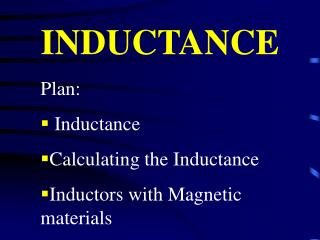DownloadDownload PresentationINDUCTANCE

# INDUCTANCE

Télécharger la présentation## INDUCTANCE

- - - - - - - - - - - - - - - - - - - - - - - - - - - E N D - - - - - - - - - - - - - - - - - - - - - - - - - - -
##### Presentation Transcript

1. INDUCTANCE • Plan: • Inductance • Calculating the Inductance • Inductors with Magnetic materials

2. Inductor • Capacitor stores energy in an electric field • Inductor stores energy in magnetic field

3. Mutual Inductance Flux in the loop 2 is proportional to current in the loop one.

4. Self Inductance A changing current not only induces emf in the nearby loops, it also induces an emf in the source loop itself.

5. Current i produces B in the interior of the coil • Changing current generates emf in the same coil.

6. Units : volt-second/ampere (Henry)

7. Calculating the Inductance NB is called flux linkage

8. A geometrical property

9. Inductance of a solenoid n is number of turns per unit length.

10. Exercise • Inductance of a closed packed coil of 400 turns is 8.0 mH. Calculate the magnetic flux through the coil when the current is 5 mA. =1 x 10-7 Wb

11. Exercise • A circular coil has a 10.3 cm radius and consists of 34 closely wound turns of wire. An externally produced magnetic field of 2.62 mT is perpendicular to the coil. • (a) If no current in the coil what is the number of flux linkages?

12. (b)When the current in the coil is 3.77 A the net flux in the coil is found to vanish. Find the inductance of the coil. L = 7.88 x 10-4H

13. Inductance of a Toroid

14. Is the current increasing or decreasing? • Ans: Decreasing

15. What is the value of inductance if rate of change of current is 25 kA/s and the emf is 17 V. • Ans: L = 6.8 x 10-4 H

16. Magnetic materials in inductors • Dielectric substance increases the capacitance • Same way, filling the magnetic material can increase the inductance.

17. m is the permeability of the material

18. Inductance increases by a factor of m

19. Inductors in series = 1 + 2

20. Inductors in parallel • Current through each inductor will add to give the total current.

21. A toroid having 5.20 cm square cross section and an inside radius of 15.3 cm has 536 turns of wire and carries a current of 810 mA. Calculate the magnetic flux through a cross section h = 5.20 cm, a = 15.3 cm, N = 536 b = (5.2 + 15.3) cm,

22. Ans : B = 1.32 x 10-6 Wb

23. Current through a 4.6 H inductor varies with time as shown. Calculate the induced emf in the time intervals

24. (a) T = 0 to t = 2 ms • Ans: 1.6 x 104 V

25. (a) T = 5 to t = 6 ms Ans: 2.3 x 104 V

26. A coaxial cable has an inner wire of radius a and an outer metal sheath with inner radius b. A current I flows down the inner wire and back through the sheath. What is the self inductance of a unit length of the cable?

27. The magnetic field in the interior of a solenoid has the value of 6.5 x 10-4 T when the solenoid is empty. When it is filled with iron, the field becomes 1.4 T. Find the average magnetic moment of iron under these conditions. Number of atoms per unit volume is given as 8.49 x 1023 atoms/m3 • Magnetic moment per atom is M = 1.11 x 106 A/m  = 1.4 B

28. Two long parallel wires, each radius a, whose centers are at a distance d apart carry equal current in opposite directions. Neglecting the flux within the wire themselves, find the inductance of a length l of such a pair of wires. . x

29. . d-a d-y d p y a x

30. An alternating current I0 cost flows down a straight wire. Which runs along the axis of a toroidal coil with rectangular cross section. The coil is connected to a resistance of R. (a) What is the emf induced in the toroid? Flux through the N turns of the toroid

31. Find the induced current in the resistor • Calculate the back emf in the coil due to this induced current.

32. In some circuits a spark occurs between the poles of the switch when the switch is opened. Yet for the switch for the circuit is closed no spark occurs. Why is this difference? • When the switch is opened, the sudden drop in B induced an emf that attempts the original current flowing. This causes a spark as the current bridges the air gap between the poles of the switch.